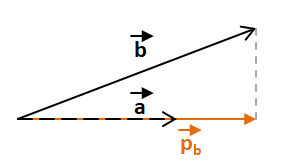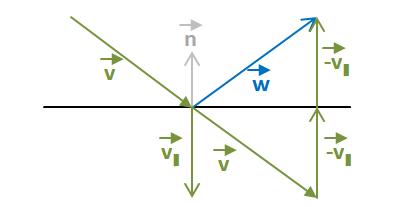﻿ Sunshine's Homepage - Vector reflection at a surface
Vector reflection at a surface (General explanation and Typescript implementation)

### Introduction

This page explains and visualizes how a vector is reflected at a surface. It is implemented in Typescript using HTML5 canvas.
Feel free to download the source code at the top of the page to check out how it works.
See below for the derivation of the required mathematics.

### Usage

Above an interactive visuation of the vector reflection is shown: The green vector v is reflected at the plane p with normal n and the result is the blue reflected vector.
The black rectangles can be clicked and dragged to modify the plane orientation and the input vector v.

### Mathematical explanation

#### Prerequisites

In the following, a vector v is denoted in bold as v. The length of v is written as |v|.
A vector that is parallel to v is written as v, a vector that is perpendicular to v is written as v.
The unit vector of v is written as vn. The dot product of v and w is written as vw.

#### DotProduct

The most essential mathematic tool that is going to be used is the dot product of two vectors, so at first a short recapitulation.
The dot product is two vectors a and b is calculated as ab = a1 * b1 + a2 * b2 ... + an * bn.
Generally, the important property of the dot product is that is can be used to calculate the angle ɑ between two vectors (because ab = |a| * |b| * cos (ɑ)).

However, another geometric property is most often even more useful:As shown in the figure above, the vector b is projected onto vector a, resulting in pb. The dot product can be used to calculate the length of the projected vector pb, i.e |pb|.
The concrete relation is as follows: The dot product is equal to the signed length of the projection of b onto a multipled by the length of a. So |pb| = (ab / |a|).
If a is a unit vector, then the dot product provides the desired result directly without division: |pb| = anb.

This fact provides an even more practical tool which is shown in the following figure:It can be used to split b into two vectors: One vector that is parallel to a which is denoted as b, the other vector that is perpendicular to a which is denoted as b.

The length of b is: |b| = anb.
So to get the actual vector b, just multiply it with an: b = (anb) * an.

Finally, getting b is straight-forward because b is the sum of b and b: b = b + b.
Thus b = b - b = b - (anb) * an.

#### Reflection

So let's come to the actual part of reflection. The following figure shows an overview of the task: A vector v shall be reflected at a plane p with normal vector n (|n| = 1). The goal is to calculate the reflected vector w of vector v.The solution is to decompose the vector v into its parallel component v and its perpendicular component v and use them in a clever way to represent vector w.The figure on the right side shows the reflection from above, but with the subtle difference that the v is negated and called v'.
This eases the understanding of the following derivation: If v' is followed and then v' is followed in reverse direction twice times, the result is w, thus:
w = v' + (- v') + (- v') = v' - 2 * v'.

As v' = -v, let's express w in terms of v and do some replacements and simplifications:
w = -v - 2 * (-v)
= -v - 2 * (-v - (-vn) * n)
= -v + 2 * (v + (-vn) * n)
= -v + 2 * v + 2 * (-vn) * n
= v + 2 * (-vn) * n
= v - 2 * (vn) * n
= v - 2 * v

#### Reflection in a nutshell:

w = v - 2 * (vn) * nThe formula w = v - 2 * (vn) * n = v - 2 * v can also be understood from a geometrical point of view.

As shown in the figure right, the vector v is appended again at the point where it hits the plane (that's legitimate as a vector only describes a direction, not a point in space).
So if v is followed from this point and afterwards v is followed in reverse direction (-v) for two times, the result is vector w.
That's it! Hope you liked it and learned something new!

Sunshine, March 2021

#### History

• 2021/03/14: Initial version.